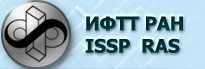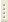06.02.2023Interacting Electrons in Normal Metals

Prof. V.T.Dolgopolov

The course is an introduction to interacting Fermi-particle physics and intended for future experimentalists. Its content is limited to fairly simple techniques and requires only knowledge of quantum mechanics at the secondary quantization level. However, the content of the course is sufficient for qualitative comprehension of the focal problems of state-of-the-art solid state physics.

Curriculum

1. Introduction. Coulomb Fermi-gas particle interaction. Kohn-Luttinger theorem. Elementary conceptions of Fermi liquid. Quasi-particles in terms of secondary quantization.
2. Ground state problem: problem statement. Perturbation theory and Brackner-Goldstone rule. Hartree-Fock approximation. Ground state energy of free electron gas.
3. Bardeen-Cooper-Schrieffer model. Effective Hamiltornian. Hartree-Fock approximation analogy. Normal quasi-particle spectrum.
4. External disturbance response (neutral systems). Test particle concept. Dynamic form-factor and density correlation. Static form-factor. Linear reaction function.
5. Calculation of density-density reaction function. Relation of density-density reaction function to compressibility of Fermi liquid and thermodynamic state density. Expression of linear reaction function by dynamic form-factor. Density-density reaction function of free Fermi gas in long-wave limit.
6. Charged Fermi liquid. Static screening.  Dynamic screening, plasma fluctuations. Relation of dielectric permeability to density-density reaction function. Screened field reaction.
7. Microscopic theories of charged system response. Calculation of dielectric permeability in Hartree-Fock approximation. Pair correlation function.
8. Calculation of dielectric permeability in random phase approximation. Relation of results to screened field reaction. Comparison of accuracy of different microscopic theories.
9. Uniform electromagnetic field response. Interaction Hamiltonian. Kubo-Greenwood formula. Thouless considerations and scaling hypothesis.
10.  Examples of practical application of studied techniques. Dielectric permeability of two-dimensional electron gas. Two-dimensional electron scattering on Coulomb centers. Temperature dependence of elastic relaxation time. Comparison to experimental results.
11. Landau Fermi liquid theory. Free energy expansion. Local quasi-particle energy. Derivation of kinetic equation.
12.  Flux density and effective quasi-particle mass. Collective modes, zero sound. Comparison of zero and first sound velocities and damping.
13. Spin polarization dependence of conductivity of two-dimensional electron systems.